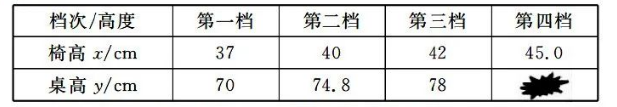(1) 在上面的表格中, 有一个数据被污染了, 则被污染的数据为
(2) 设课桌的高度为 $y(\mathrm{~cm})$, 椅子的高度为 $x(\mathrm{~cm}$ ), 求 $y$ 与 $x$ 的函数关系式 (不必写出自变量 的取值范围).
(3) 小明放学回到家, 又测量了家里的写字台的高度为 $77 \mathrm{~cm}$, 登子的高度为 $41 \mathrm{~cm}$, 请你判断 小明家里的写字台与凳子是否符合科学设计, 并说明理由.

【答案】 (1) $82.8$
(2) $y=16 x+10.8$
(3) 不配套

$\because 76.4 \neq 77$
$\therefore$ 不配套